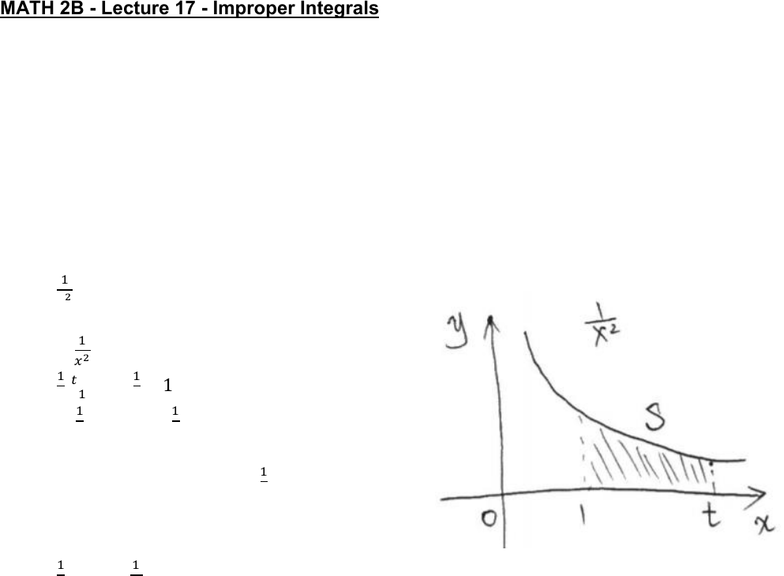Class Notes (1,100,000)
US (460,000)
UC-Irvine (10,000)
MATH (1,000)
MATH 2B (600)
Lecture 17

# MATH 2B Lecture 17: Improper IntegralsPremium

Department
Mathematics
Course Code
MATH 2B
Professor
ERJAEE, G.
Lecture
17

This preview shows half of the first page. to view the full 3 pages of the document.02/13/2019
MATH 2B - Lecture 17 - Improper Integrals
We know the definite integral usually has a function f(x) that is defined on a finite
interval [a,b]. Now we generalize the concept by considering two cases:
1. Infinite Intervals
2. Discontinuous Integrands
Infinite Intervals
Example:
Consider the region bounded by  
, on the interval [1,t]
 
 F.T.C Part II
 
 
We note that   
is always less
than 1
If we take 
, we have 
  

  
   
This tells us that, in the case of 
, the region s has infinite length, but the area
of this region is finite 
  
This is the first type of improper integral
a) If
 exists for every number   , then
 



b) If
 exists for every number   , then
  



If the improper integrals exists and gives us a finite number, then
we say the improper integral is convergent, otherwise it is divergent
c) If
 and
  are convergent, then we can define
   

 